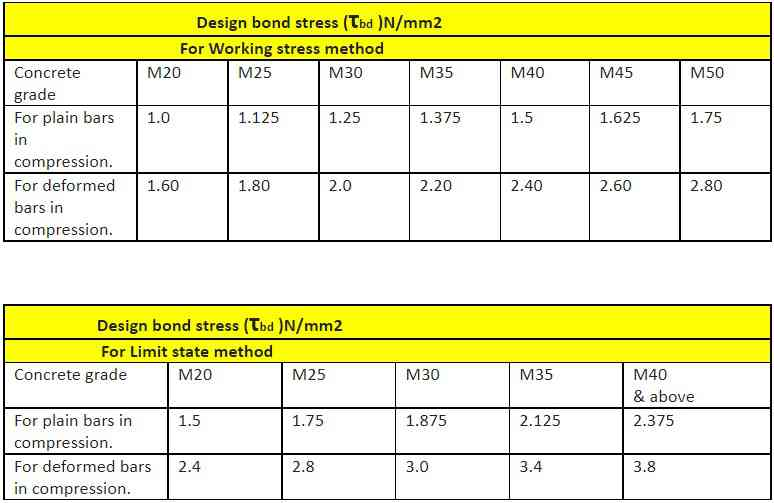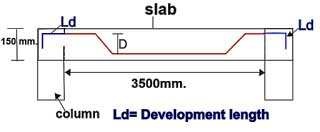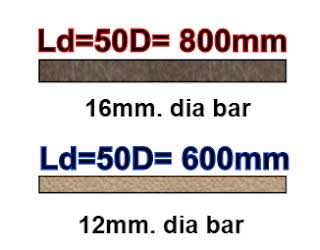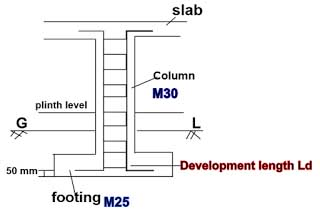## How to Calculate Development Length for Different Grades of Concrete As Per IS 456

In this Article today we will talk about the How to Calculate Development Length | Development Length of Rebar | Development Length of Reinforcement bar | Rebar Development Length Table | Rebar Development Length Calculator | Development Length Formula | Concrete Rebar Calculations | Rebar Lap Splice Length | Development Length as per IS 456

## How to Calculate Development Length of Steel Reinforcement:

As per IS-456: 2000 clause 26.2.1, the development length Ld is given by

In the IS-code 456, it is mentioned that the value of bond stress should be increased by 60% for high yield strength deformed bars, & it should be further increased by 25% for the calculation of development length in compression. Rebar Development Length Calculator

I have made a table of design bond stress for the limit state method & working stress method as follows.

Note: For the compression, I have further increased the value of design bond stress by 25% and made a table. Concrete Rebar CalculationsNow, let us consider Two examples to calculate the development length for different grades of concrete.

## Example – 1

### Given data:

Calculate the development length for rebar in tension, by limit state method for the below-given data.

Reinforcement bar = Fe500 (σs = 500 )

Diameter of bar = 16mm. (∅ )

The development length Ld =  [ ∅ × σs ÷  4 × τbd]

Ld = [16 × 500 ÷ 4 × 2.40]

( From the above-given table, τbd = 2.40 N/mm2 for M30 in tension, for the limit state. )

= [8000 ÷ 9.6]

Ld = 833mm. Rebar Lap Splice Length

## Example – 2

### Given data:

Calculate the development length for rebar in compression, by working stress method for the below-given data.

Reinforcement bar = Fe415 (σs = 415 )

Diameter of bar = 12mm. (∅ )

The development length Ld =  [ ∅ × σs ÷  4 × τbd]

Ld = [12 × 415 ÷ 4 × 1.8]

( From the above-given table, τbd = 1.8 N/mm2 for M25 in compression, for the working stress method. )

= [4980 ÷ 7.2]

Ld  = 692mm.

## FAQs

### Q. What is the development length of steel bars?The length of the reinforcement bar that is extended in the concrete, at the junction point of the two RCC members, so that the member won’t get slipped away is called the development length.

This length is provided to transfer the maximum stress to the surrounding concrete to such a length, where the holding stress becomes zero at the discontinuous end.

### Q. What are the factors that affect the development length?

The factors that affect the development length are,

The higher the grade of concrete, the lesser will be the development length.

#### 2. Diameter of the reinforcement bar:

The development length of the rebar increases with the increase in the diameter of a bar.#### 3. Yield strength & stress on the different grades of steel rebars:

The higher the stress in a bar at the section considered at design load, the more will be the development length.

#### 4. Type of structural member:

The development length will be 25% more in the compression member than in the tension member.

#### 5. Calculation method for designing:

In the limit state method of designing, the development length will be less when compared to the designing of the same structure by using the working stress method.

### Q. What happens if we don’t provide sufficient Development Length?

If we provide insufficient development length, the structure may slip away at the joints or junction point of two RCC members. The lesser development length ensures a lack of grip or holding property to safely transfer the designed stress to the succeeding structural member, thus resulting in a failure of an RCC member at the structural joints.

### Q. What is the common mistake while calculating the development length?As you can observe in the above drawing, the development length is provided in the footings for the reinforcement bars coming from the column. Here, the grade of concrete used in the column is M30, and the M25 grade is used in casting the footings.

The value of design bond stress (τbd ) changes, according to the grade of concrete used in casting the concrete members.

In the above-given example, you have to consider the concrete grade (M25) of the footing, to evaluate the development length. The reason is, the development length of the bar is anchored in the footings, although the bar is extended from the column into the footings.

Considering the M30 grade of the concrete used in a column for the calculation purpose is a common mistake, that should be avoided to get an accurate development length.

## Conclusion:

Full article on How to Calculate Development Length | Development Length of Rebar | Development Length of Reinforcement bar | Rebar Development Length Table | Rebar Development Length Calculator | Development Length Formula | Concrete Rebar Calculations | Rebar Lap Splice Length | Development Length as per IS 456. Thank you for the full reading of this article in “The Civil Engineering” platform in English. If you find this post helpful, then help others by sharing it on social media. If any formula of BBS is missing from this article please tell me in comments.

1.Oseigho oghenemena
2.Samson from kenya